## Rayleigh-Debye-Gans approximation (RDG)

In a large number of important cases the relative refractive index of particles is close to 1. Such particles are called optically soft'', and the corresponding approximation could be named that of optically soft particles. This approximation is in particular well applicable to scattering of X-rays and neutrons [56,211,238]. The condition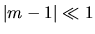is not sufficient for development of the theory since an important role is also played by the ratio of the size to the wavelength and the phase shift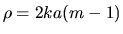. The RDG approximation is applicable when two conditions are satisfied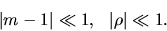(1)

For the theory based on the assumption (1), there are a set of the names: Rayleigh-Gans , Rayleigh-Debye [274,397], Rayleigh-Gans-Born , Rayleigh-Gans-Debye [78,144,409,410], Rayleigh-Gans-Rokard , Rayleigh-Debye-Gans (RDG) [100,462], Born (or the first Born) [56,135] approximation. We believe that the term RDG is the most suitable for the following reasons. The basic ideas of the method including the derivation of successive approximations from the integral equation for the scattered field were formulated by Rayleigh in 1881 in the paper , and the expression for the scattering cross-section for a sphere was obtained by him in 1914 . Independently of Rayleigh, in 1915 Debye  derived the general expression for the intensity of X-rays scattered by an ensemble of randomly oriented particles. Later the approach was applied by Debye to scattering by polymer solutions [200,202] which was very important for development of this field [26,130,274]. The contribution of Gans is of course essentially smaller than those of Rayleigh and Debye as in his paper  he actually repeated the Rayleigh result for a sphere  (in the third time the same results was obtained by Rokard, see , p.249). An analog of the Rayleigh iterations in the quantum mechanics was developed in 1926 by M. Born . This approach became very popular one in this field, and the name of Born is rightfully connected with this approximation. The name of Gans is reasonable to leave in the term the RDG theory just for the reason that due to van de Hulst  the term Rayleigh-Gans approximation became widespread, although he did not consider this term to be a good one.

The RDG approximation can be obtained in several ways [25,124,274,441]. For instance, one can use the general integral relation expressing the field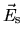scattered in the directionthrough the integral containing the tensor Green function for the vector Helmholtz equationand the field inside the particle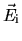, and make the latter equal to the field of the incident wave. Another way is based on the physical interpretation of scattering as a result of interference of the fields of independent dipoles excited by the incident wave in the particle volume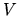. In both cases the scattered field can be represented as(2)

with the vector amplitude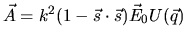, wherein the scattering vector with the module,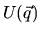is the Fourier transform of the dielectric permittivity tensor (normalized by the volume of polarizability of an optically soft particle,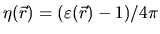). For homogeneous particles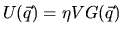, where the interference function is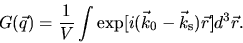(3)

Thus, the polarization characteristics of this approximation do not differ from those of the Rayleigh approximation for optically soft particles, and the angular ones differ only by the interference function.

The interference functions for particles of several shapes can be obtained in the form of simple analytical expressions. Rayleigh got the formula for a sphere in the form of a series in  and using the cylindrical functions of the order 3/2 in . He also derived the formula for an infinitely long cylinder in the case of normal incidence . Finite cylinders were considered in the paper  and in the book of van de Hulst , prisms in [82,364], ellipsoids in the papers of A.L. Patterson in 1939  (Kerker  at p.484 wrongly cites the paper  as the first one). For spheroids, the formula for the interference function was obtained in [237,238] and later in [426,428,447]. The RDG approximation can be easily generalized for inhomogeneous particles, in particular with the spherical symmetry of inhomogeneity [11,135,216], and for particles of arbitrary shape with the rotational symmetry . The tables of the interference functions see in [226,274]. The papers [274,444] suggest a small modification of the RDG where the wavenumber inside the particle takes into account the refractive index.

As one usually measures the intensity of scattered light from an ensemble of particles with a random orientation, the averaged square of the interference functioncalled the form-factor or Debye scattering form-factor (sometimes it is written simply as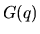) has the practical interest. A detailed literature analysis of calculations of the form-factors for different models including polydisperse ensembles and polymer solutions is given in , and here we just list some investigations in this direction [82, 149, 150, 151, 152, 156, 197, 206, 225, 238, 245, 271, 274, 294, 296, 297, 298, 299, 335, 363, 364, 365, 366, 367, 378, 379, 381, 397, 398, 402, 426, 428, 445, 446, 465, 479]. The accuracy of the RDG approximation was estimated for spheres [197,236,274,275,475], cylinders [171,438] and spheroids [144,145,297,412,475].

For anisotropic particles, the RDG theory was built in the assumption that the induced elementary dipoles are the product of the optical polarizability tensor and the incident field. In Eq. (2) the vector amplitude is not parallel to the incident field, and hence to describe the angular dependence of scattering one needs four Debye form-factors corresponding to four combinations of the polarizator and analyzator states relative to the planes of incidence and scattering (, where the subscripts v and h have the common sense  and for a system with the random orientation). Scattering by anisotropic spheres, cylinders, discs and spheroids was considered in [94, 96, 100, 130, 246, 255, 256, 261, 262, 375, 399, 406, 407, 408, 409, 410, 411, 415, 423, 479]. After the pioneer work  a large attention in the literature on polymers is paid to the scattering by specific anisotropic structures - so called spherulites [4,5,403,429,456].

The extinction cross-section in the RDG approximation is proportional to the volume and in general case does not differ in form from the extinction cross-section in the Rayleigh approximation. The scattering cross-section should be found from integration of the intensity over all solid angles, since the optical theorem in the RDG approximation gives either the extinction cross-section or 0 (for non-absorbing particles). The first calculations of the RDG scattering cross-section for the spheroidal model of bacteria cells were performed by in 1961 by A.L. Koch . A systematic study of the integral functions in the method of the turbidity spectrum of spheroidal particles (the scattering cross-section and wave exponent  was made in [100,104,116,118,119,120]. These works contain the general integral representations, results of numerical computations and asymptotic analytic expressions found for the region of small sizes and the intermediate region between the RDG and anomalous diffraction theories as well as in the cases of weak and strong eccentricity. Later the analysis of the integral characteristics of RDG-spheroids was also made in [44,49,53,75].To the top
Created by V.I.